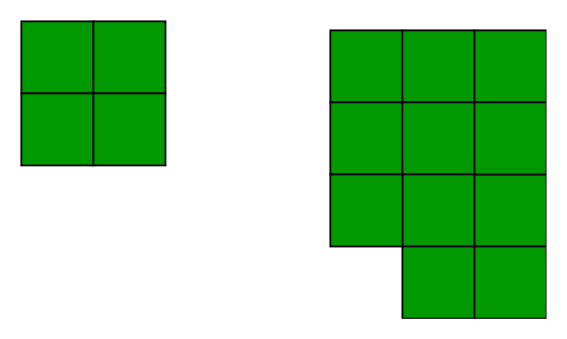# Minimum Perimeter of n blocks

We are given n blocks of size 1 x 1, we need to find the minimum perimeter of the grid made by these blocks.

Examples :

```Input : n = 4
Output : 8
Minimum possible perimeter with 4 blocks
is 8. See below explanation.

Input : n = 11
Output : 14
The square grid of above examples would be as```

## Recommended: Please try your approach on {IDE} first, before moving on to the solution.

Let us take an example to see a pattern. Let us say that we have 4 blocks, following are different possibilities

```
+--+--+--+--+
|  |  |  |  |  Perimeter = 10
+--+--+--+--+

+--+--+--+
|  |  |  |     Perimeter = 10
+--+--+--+
|  |
+--+

+--+--+--+
|  |  |  |     Perimeter = 10
+--+--+--+
|  |
+--+

+--+--+
|  |  |        Perimeter = 8
+--+--+
|  |  |
+--+--+
```

If we do some examples using pen and paper, we can notice that the perimeter becomes minimum when the shape formed is closest to a square. The reason for this is, we want maximum sides of blocks to face inside the shape so that perimeter of the shape becomes minimum.

If the Number of blocks is a perfect square then the perimeter would simply be 4*sqrt(n).
But, if the Number of blocks is not a perfect square root then we calculate number of rows and columns closest to square root. After arranging the blocks in a rectangular we still have blocks left then we will simply add 2 to the perimeter because only 2 extra side would be left.
The implementation of the above idea is given below.

## C++

 `// CPP program to find minimum  ` `// perimeter using n blocks. ` `#include ` `using` `namespace` `std; ` ` `  `int` `minPerimeter(``int` `n) ` `{ ` `    ``int` `l = ``sqrt``(n); ` `    ``int` `sq = l * l; ` ` `  `    ``// if n is a perfect square ` `    ``if` `(sq == n)  ` `        ``return` `l * 4; ` `    ``else` `    ``{ ` `        ``// Number of rows  ` `        ``long` `long` `int` `row = n / l;  ` ` `  `        ``// perimeter of the  ` `        ``// rectangular grid  ` `        ``long` `long` `int` `perimeter  ` `                      ``= 2 * (l + row);  ` ` `  `        ``// if there are blocks left  ` `        ``if` `(n % l != 0)  ` `            ``perimeter += 2; ` `        ``return` `perimeter; ` `    ``} ` `} ` ` `  `// Driver code ` `int` `main() ` `{ ` `    ``int` `n = 10; ` `    ``cout << minPerimeter(n); ` `    ``return` `0; ` `} `

## Java

 `// JAVA Code to find minimum  ` `// perimeter using n blocks ` `import` `java.util.*; ` ` `  `class` `GFG  ` `{ ` `    ``public` `static` `long` `minPerimeter(``int` `n) ` `    ``{ ` `        ``int` `l = (``int``) Math.sqrt(n); ` `        ``int` `sq = l * l; ` `     `  `        ``// if n is a perfect square ` `        ``if` `(sq == n)  ` `            ``return` `l * ``4``; ` `        ``else` `        ``{ ` `            ``// Number of rows  ` `            ``long` `row = n / l;  ` `     `  `            ``// perimeter of the  ` `            ``// rectangular grid  ` `            ``long` `perimeter  ` `                  ``= ``2` `* (l + row);  ` `     `  `            ``// if there are blocks left  ` `            ``if` `(n % l != ``0``)  ` `                ``perimeter += ``2``; ` `            ``return` `perimeter; ` `        ``} ` `    ``} ` `     `  `    ``// Driver code ` `    ``public` `static` `void` `main(String[] args)  ` `    ``{ ` `        ``int` `n = ``10``; ` `        ``System.out.println(minPerimeter(n)); ` `    ``} ` `} ` ` `  `// This code is contributed by Arnav Kr. Mandal `

## Python3

 `# Python3 program to find minimum  ` `# perimeter using n blocks. ` `import` `math ` ` `  `def` `minPerimeter(n): ` `    ``l ``=` `math.sqrt(n) ` `    ``sq ``=` `l ``*` `l ` `  `  `    ``# if n is a perfect square ` `    ``if` `(sq ``=``=` `n):  ` `        ``return` `l ``*` `4` `    ``else` `: ` `        ``# Number of rows  ` `        ``row ``=` `n ``/` `l ` `  `  `        ``# perimeter of the  ` `        ``# rectangular grid  ` `        ``perimeter ``=` `2` `*` `(l ``+` `row) ` `                       `  `        ``# if there are blocks left  ` `        ``if` `(n ``%` `l !``=` `0``):  ` `            ``perimeter ``+``=` `2` `        ``return` `perimeter ` ` `  `# Driver code ` `n ``=` `10` `print``(``int``(minPerimeter(n))) ` ` `  `# This code is contributed by  ` `# Prasad Kshirsagar `

## C#

 `// C# Code to find minimum  ` `// perimeter using n blocks ` `using` `System; ` ` `  `class` `GFG  ` `{ ` `    ``public` `static` `long` `minPerimeter(``int` `n) ` `    ``{ ` `        ``int` `l = (``int``) Math.Sqrt(n); ` `        ``int` `sq = l * l; ` `     `  `        ``// if n is a perfect square ` `        ``if` `(sq == n)  ` `            ``return` `l * 4; ` `        ``else` `        ``{ ` `            ``// Number of rows  ` `            ``long` `row = n / l;  ` `         `  `            ``// perimeter of the  ` `            ``// rectangular grid  ` `            ``long` `perimeter ` `                  ``= 2 * (l + row);  ` `     `  `            ``// if there are blocks left  ` `            ``if` `(n % l != 0)  ` `                ``perimeter += 2; ` `            ``return` `perimeter; ` `        ``} ` `    ``} ` `     `  `    ``// Driver code ` `    ``public` `static` `void` `Main()  ` `    ``{ ` `        ``int` `n = 10; ` `        ``Console.Write(minPerimeter(n)); ` `    ``} ` `} ` ` `  `// This code is contributed by nitin mittal `

## PHP

 ` `

Output :

```14
```

This article is contributed by Sarthak Kohli. If you like GeeksforGeeks and would like to contribute, you can also write an article using contribute.geeksforgeeks.org or mail your article to contribute@geeksforgeeks.org. See your article appearing on the GeeksforGeeks main page and help other Geeks.

My Personal Notes arrow_drop_up

Article Tags :
Practice Tags :

Be the First to upvote.

Please write to us at contribute@geeksforgeeks.org to report any issue with the above content.